# Alouette Reservoir Kokanee Size-at-Age 2019

The suggested citation for this analytic appendix is:

Thorley, J.L. (2020) Alouette Reservoir Kokanee Size-at-Age 2019. A Poisson Consulting Analysis Appendix. URL: https://www.poissonconsulting.ca/f/1304398440.

## Background

The Kokanee age structure population analysis (ALUMON #6) is a multi-year study to address potential impacts of reservoir operations on the reservoirs’ kokanee (Oncorhynchus nerka) population (Andrusak 2016).

The management question addressed in the current analysis is whether:

1. The size-at-age of the kokanee population remains stable, or decreases with time once the standing crop has stabilized with the annual addition of fertilizer.

## Methods

### Data Preparation

The gillnetting and productivity data were provided by BC Ministry of Forests, Lands and Natural Resource Operations.

#### Size-at-Age

The data used for size-at-age analysis were the lengths of age-3 Kokanee caught during gillnetting surveys.

During the survey data preparation it was assumed that:

• Standing crop stabilized in 2003.
• Only fish that were netted in September and October had indicative lengths.
• The productivity was the N:P ratio from 2008-2013 of 7.45 multiplied by the nutrient with the lower value in the ratio (P).

### Statistical Analysis

Model parameters were estimated using Bayesian methods. The estimates were produced using JAGS (Plummer 2015). For additional information on Bayesian estimation the reader is referred to McElreath (2016).

Unless stated otherwise, the Bayesian analyses used weakly informative normal and half-normal prior distributions (Gelman, Simpson, and Betancourt 2017). The posterior distributions were estimated from 1500 Markov Chain Monte Carlo (MCMC) samples thinned from the second halves of 3 chains (Kery and Schaub 2011, 38–40). Model convergence was confirmed by ensuring that the potential scale reduction factor $$\hat{R} \leq 1.05$$ (Kery and Schaub 2011, 40) and the effective sample size (Brooks et al. 2011) $$\textrm{ESS} \geq 150$$ for each of the monitored parameters (Kery and Schaub 2011, 61).

The parameters are summarised in terms of the point estimate, standard deviation (sd), the z-score, lower and upper 95% confidence/credible limits (CLs) and the p-value (Kery and Schaub 2011, 37, 42). The estimate is the median (50th percentile) of the MCMC samples, the z-score is $$\mathrm{mean}/\mathrm{sd}$$ and the 95% CLs are the 2.5th and 97.5th percentiles. A p-value of 0.05 indicates that the lower or upper 95% CL is 0.

The results are displayed graphically by plotting the modeled relationships between particular variables and the response(s) with the remaining variables held constant. In general, continuous and discrete fixed variables are held constant at their mean and first level values, respectively, while random variables are held constant at their typical values (expected values of the underlying hyperdistributions) (Kery and Schaub 2011, 77–82). When informative the influence of particular variables is expressed in terms of the effect size (i.e., percent change in the response variable) with 95% confidence/credible intervals (CIs, Bradford, Korman, and Higgins 2005).

The analyses were implemented using R version 3.6.2 (R Core Team 2019) and the mbr family of packages.

### Model Descriptions

#### Size-at-Age

The fork length of age-3 Kokanee was analysed using a hierarchical Bayesian generalized mixed effects model.

Key assumptions of the model include:

• Length varies with year and day of the year.
• Length varies randomly with year and location within year.
• The residual variation in length is log-normally distributed.

Preliminary analysis indicated that productivity and type of net set were not significant predictors of the length of age-3 Kokanee.

### Model Templates

#### Size-at-Age

.model{
bLength ~ dnorm(5, 1^-2)
bYear ~ dnorm(0, 1^-2)
bDayte ~ dnorm(0, 1^-2)

bHabitat <- 0
for (i in 2:nHabitat) {
bHabitat[i] ~ dnorm(0, 1^-2)
}

sLengthAnnual ~ dnorm(0, 1^-2) T(0,)
for (i in 1:nAnnual) {
bAnnual[i] ~ dnorm(0, sLengthAnnual^-2)
}

sLocationAnnual ~ dnorm(0, 1^-2) T(0,)
for (i in 1:nLocation) {
for(j in 1:nAnnual) {
bLocationAnnual[i, j] ~ dnorm(0, sLocationAnnual^-2)
}
}

sLength ~ dnorm(0, 1^-2) T(0,)

for(i in 1:length(Length)){
log(eLength[i]) <- bLength + bHabitat[Habitat[i]] + bYear * Year[i] + bDayte * Dayte[i] + bAnnual[Annual[i]] + bLocationAnnual[Location[i], Annual[i]]

Length[i] ~ dlnorm(log(eLength[i]), sLength^-2)
}
..

Block 1. Model description.

## Results

### Tables

#### Size-at-Age

Table 1. Parameter descriptions.

Parameter Description
Annual[i] Year of capture of ith fish (as a discrete variable)
bAnnual[i] Effect of ith Annual on bLength
bDayte Effect of Dayte on bLength
bHabitat Effect of Habitat on bLength
bLength Intercept for log(eLength)
bLocationAnnual[i, j] Effect of ith Location in jth Annual on bLength
bYear Effect of Year on bLength
Dayte[i] Day of the year of capture of ith fish
eLength[i] Expected Length of ith fish
Habitat[i] Habitat of capture of ith fish (Pelagic vs Nearshore)
Length[i] Measured fork length of ith fish
Location[i] Location of capture of ith fish
sLength SD of residual variation in Length about log(eLength)
sLengthAnnual SD of bAnnual
sLocationAnnual SD of bLocationAnnual
Year[i] Year of capture of ith fish (as a continuous variable)

Table 2. Model coefficients.

term estimate sd zscore lower upper pvalue
bDayte -0.0016044 0.0005773 -2.790751 -0.0026885 -0.0004505 0.0053
bHabitat 0.0286835 0.0126052 2.316889 0.0050427 0.0540167 0.0187
bLength 5.5484459 0.0133073 416.914040 5.5206369 5.5739654 0.0007
bYear -0.0044153 0.0026635 -1.648343 -0.0094982 0.0010432 0.1053
sLength 0.0515231 0.0011101 46.465559 0.0495159 0.0539029 0.0007
sLengthAnnual 0.0446892 0.0107811 4.294392 0.0297489 0.0718660 0.0007
sLocationAnnual 0.0188552 0.0034137 5.585832 0.0129948 0.0262084 0.0007

Table 3. Model summary.

n K nchains niters nthin ess rhat converged
1136 7 3 500 50 286 1.004 TRUE

### Figures

#### Size-at-Age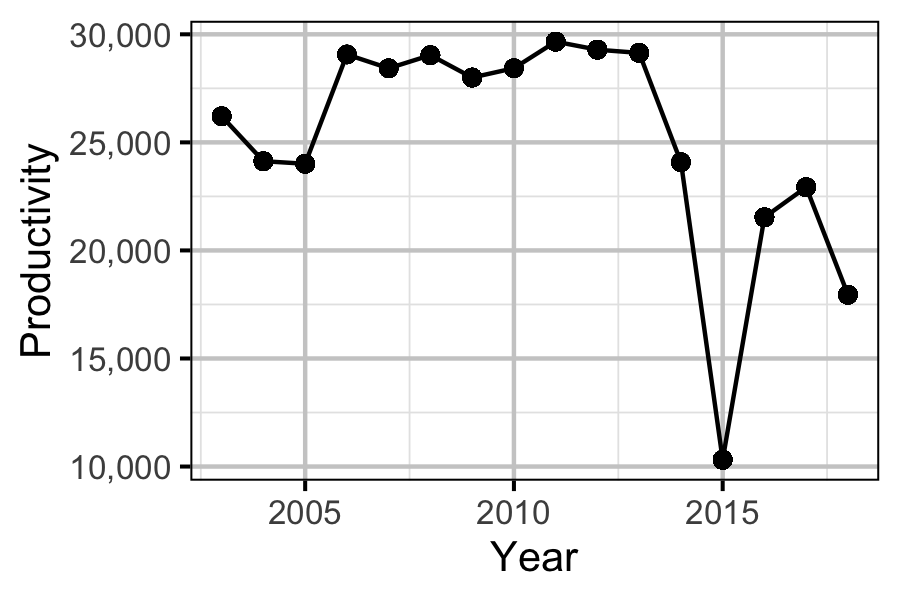Figure 1. Calculated productivity (scaled N:P ratio) by year.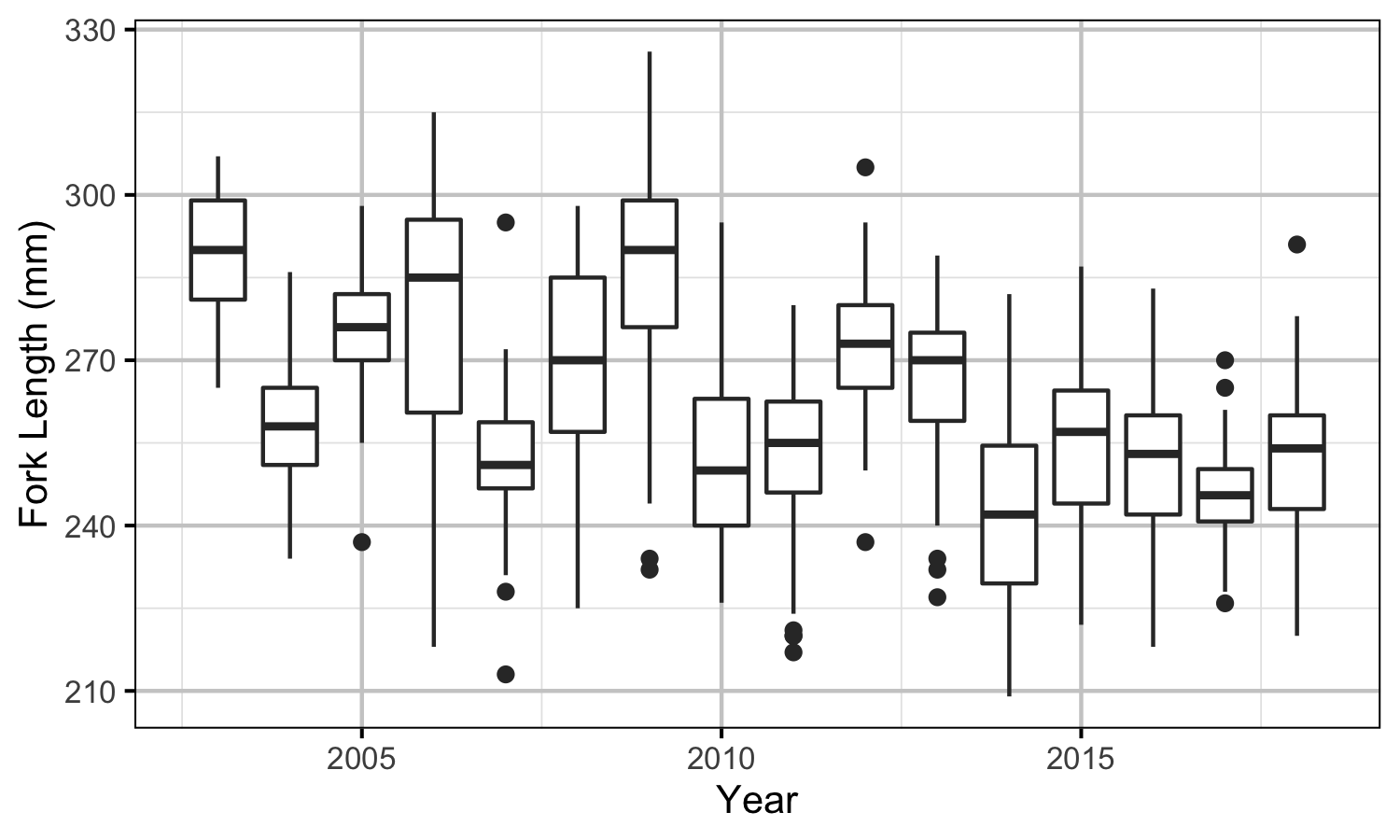Figure 2. A boxplot of the measured lengths of age-3 Kokanee by year. The data from 2003 to 2007 are from near-shore netsets while the data from 2008 onwards are from pelagic netsets.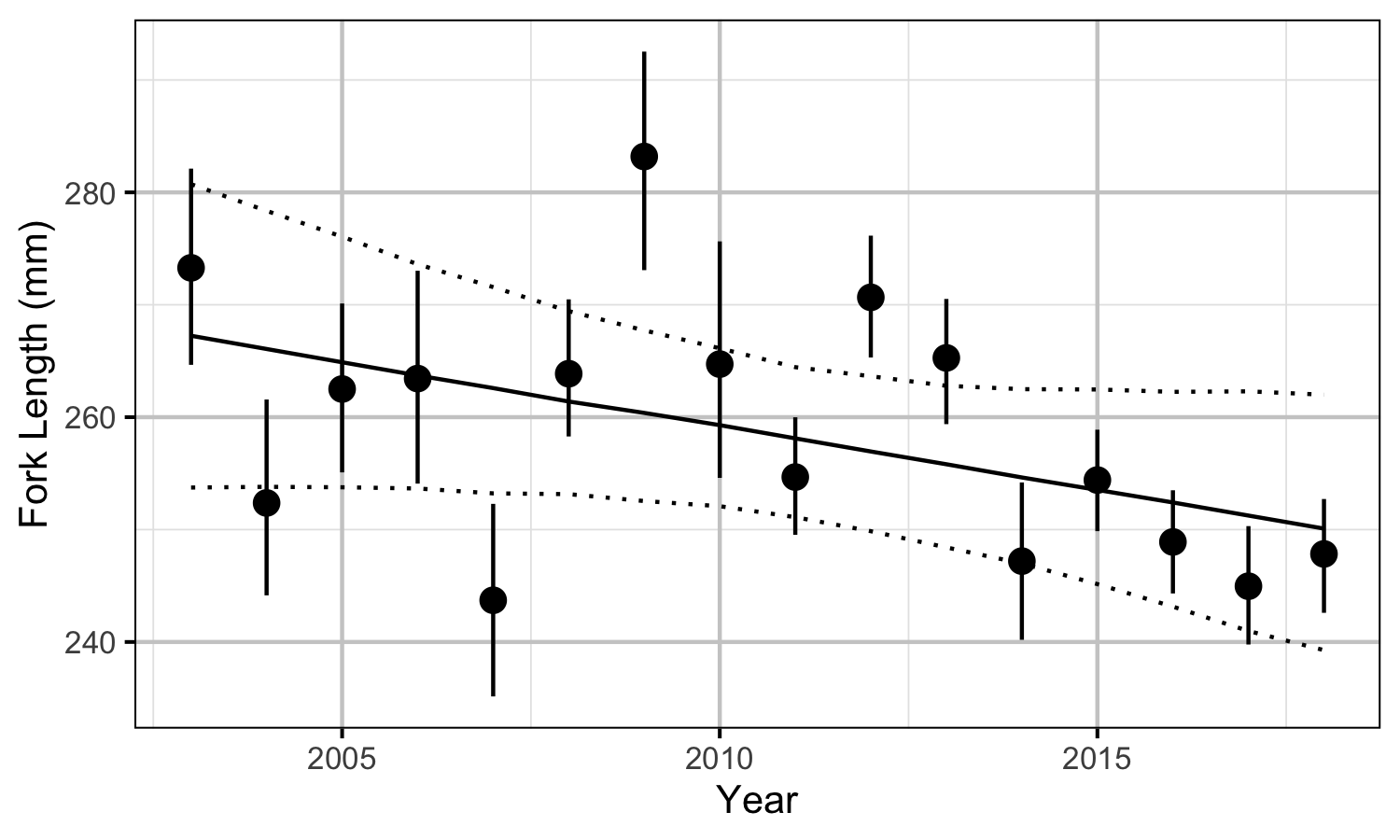Figure 3. Estimated length of age-3 pelagic Kokanee by yearly trend with annual variation (with 95% CIs).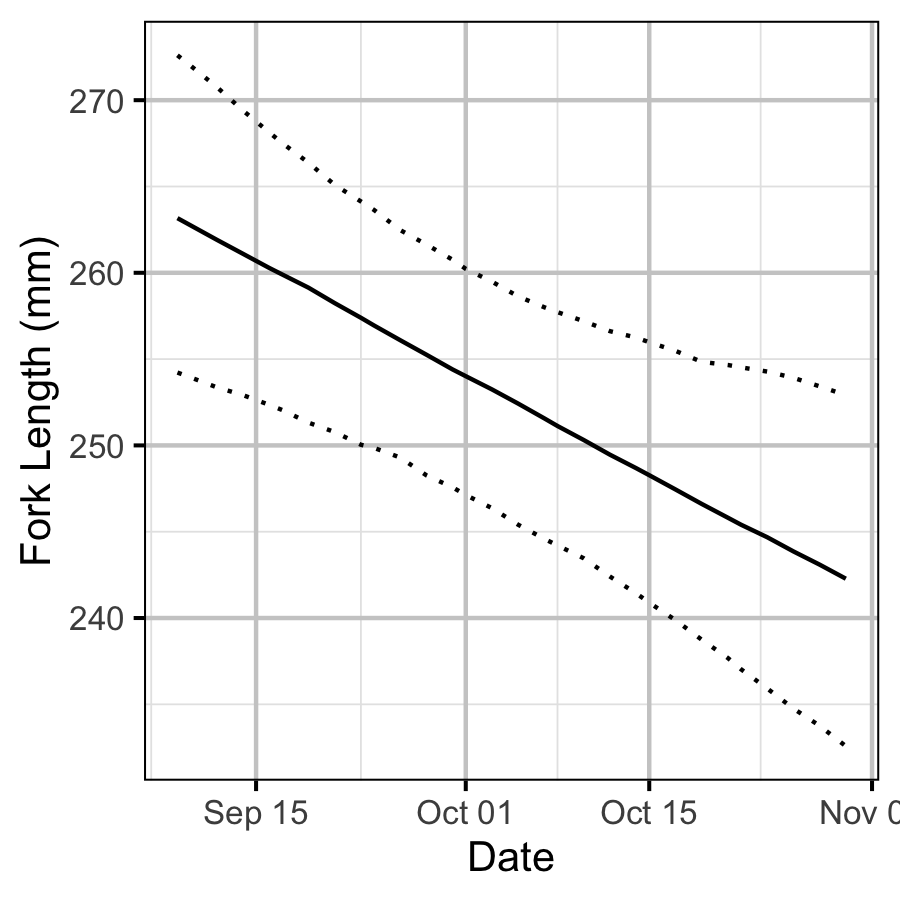Figure 4. Estimated length of age-3 pelagic Kokanee by date (with 95% CIs).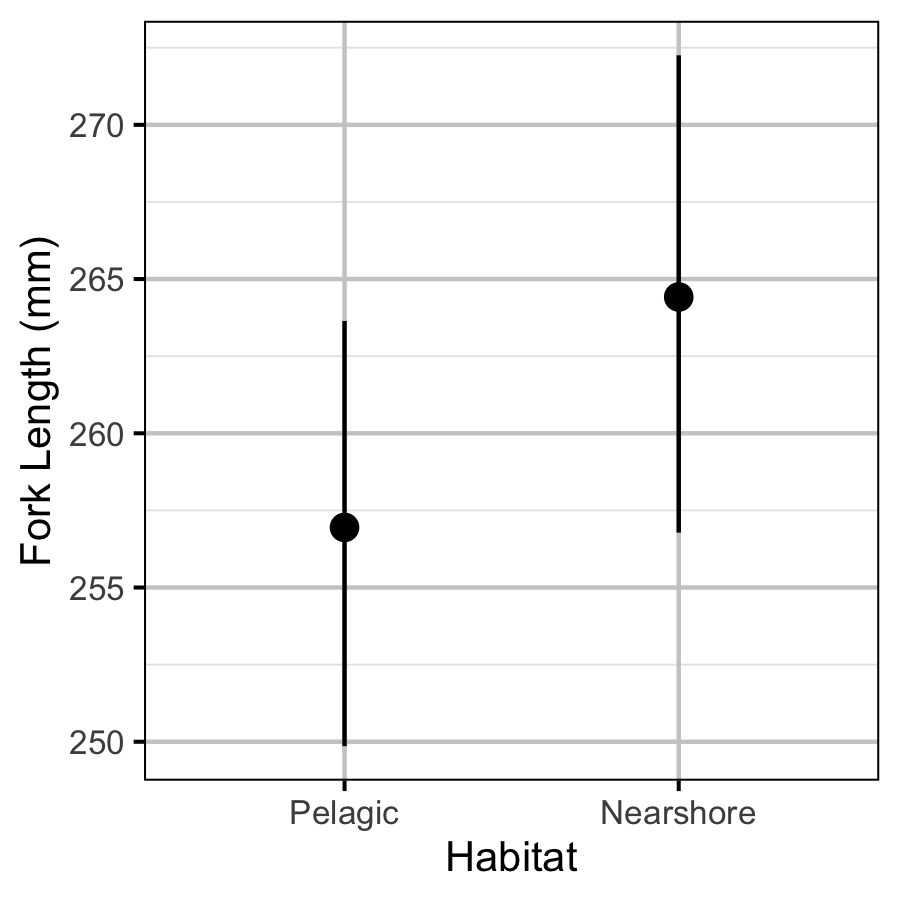Figure 5. Estimated length of age-3 Kokanee by habitat (with 95% CIs).

## Acknowledgements

The organisations and individuals whose contributions have made this analysis report possible include:

• Ministry of Forests, Lands and Natural Resource Operations (MFLNRO)
• Jen Sarchuk
• Shannon Harris
• Greg Andrusak
• Redfish Consulting
• Harvey Andrusak
• Poisson Consulting
• Robyn Irvine
• Mauricio Campos
• Patrick Hogan